# High School Chemistry : Using Boyle's Law

## Example Questions

### Example Question #22 : Phases Of Matter

An ideal gas exerts a pressure of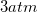in a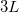container. The container is at a temperature of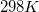.

What will be the final pressure if the volume of the container changes to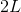?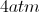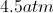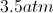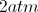Explanation:

Since the volume of the gas is the only variable that has changed, we can use Boyle's law in order to find the final pressure. Since pressure and volume are on the same side of the ideal gas law, they are inversely proportional to one another. In other words, as one increases, the other will decrease, and vice versa.

Boyle's law can be written as follows: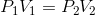Use the given volumes and the initial pressure to solve for the final pressure.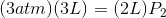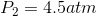### Example Question #23 : Phases Of Matter

What law is the following formula?Ideal gas law

Charles's law

Boyle's law

Combined gas law

Gay-Lussac's law

Boyle's law

Explanation:

Boyle's law relates the pressure and volume of a system, which are inversely proportional to one another. When the parameters of a system change, Boyle's law helps us anticipate the effect the changes have on pressure and volume.Charles's law relates temperature and volume: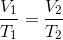Gay-Lussac's law relates temperature and pressure: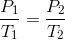The combined gas law takes Boyle's, Charles's, and Gay-Lussac's law and combines it into one law: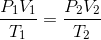The ideal gas law relates temperature, pressure, volume, and moles in coordination with the ideal gas constant: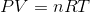### Example Question #24 : Phases Of Matter

A helium balloon has a volume of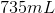when it is at ground level. The balloon is transported to an elevation of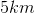, where the pressure is only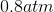. At this altitude the gas occupies a volume of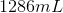. Assuming the temperature has remained the same, what was the ground level pressure?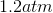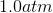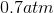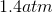Explanation:

To solve this question we will need to use Boyle's law: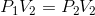We are given the final pressure and volume, along with the initial volume. Using these values, we can calculate the initial pressure.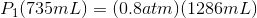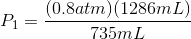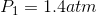Note that the pressure at sea level is equal to. A pressure greater thansimply indicates that the ground level is below sea level at this point.

### Example Question #24 : Phases Of Matter

The graph depicted here represents which of the gas laws?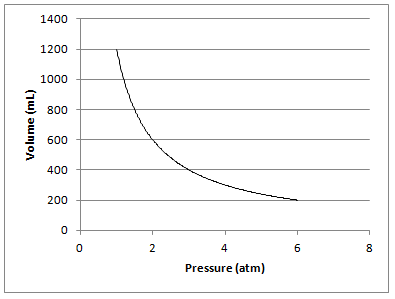Boyle's law

Charles's law

Gay-Lussac's law

Hund's law

Boyle's law

Explanation:

The graph shows that there is an inverse relationship between the volume and pressure of a gas, when kept at a constant temperature. This was described by Robert Boyle and can be represented mathematically as Boyle's law:Gay-Lussac's law shows the relationship between pressure and temperature. Charles's law shows the relationship between volume and temperature. Hund's rule (Hund's law) is not related to gases, and states that electron orbitals of an element will be filled with single electrons before any electrons will form pairs within a single orbital.

### Example Question #1 : Using Boyle's Law

A sample of oxygen gas has a volume of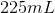when its pressure is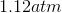. What will the volume of the gas be at a pressure of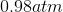if the temperature remains constant?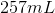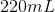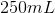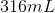Explanation:

To solve this question we will need to use Boyle's law:We are given the initial pressure and volume, along with the final pressure. Using these values, we can calculate the final volume.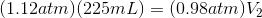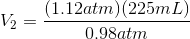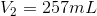### Example Question #1 : Using Boyle's Law

A gas is initially in a 5L piston with a pressure of 1atm.

If pressure changes to 3.5atm by moving the piston down, what is new volume?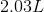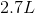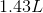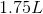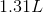Explanation:

Use Boyle's Law: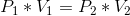Plug in known values and solve for final volume.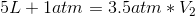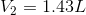### Example Question #1 : Using Boyle's Law

A balloon of volume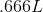at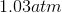is placed in a pressure chamber where the pressure becomes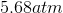, determine the new volume.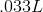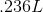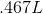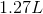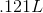Explanation:

Use Boyle's law and plug in appropriate parameters: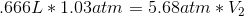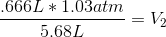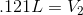### Example Question #1 : Using Boyle's Law

A gas in a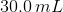container is at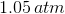is compressed to a volume of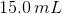. What is the new pressure of the container?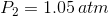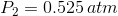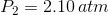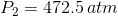Explanation:

Boyle's Law is:The initial volume (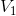) and pressure (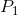) of the gas is given. The volume changes to a new volume (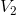). Our goal is to find the new pressure (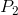). Solving for the new pressure gives: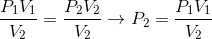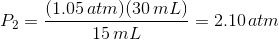Notice the answer has 3 significant figures.

### All High School Chemistry Resources